## Find the solution set of the following equations: 2y=2x+7 X=y+2

Question

Find the solution set of the following equations:

2y=2x+7

X=y+2

in progress 0
6 months 2021-07-22T15:36:40+00:00 1 Answers 5 views 0

The system of equations has no solution.

Step-by-step explanation:

Given the system of equations

2y = 2x+7

x = y+2

solving the system of equations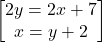Arrange equation variables for elimination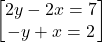Multiply -y+x=2 by 2:  -2y+2x=4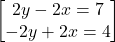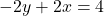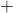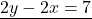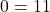so the system of equations becomes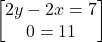0 = 11 is false, therefore the system of equations has no solution.

Thus,

No Solution!